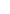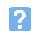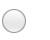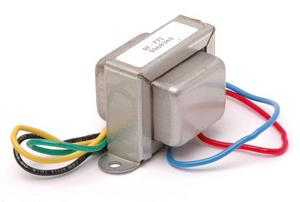## Alternating current and voltaage1. A sine wave has a periodic time of 0.25 ms. Which one of the following gives the frequency of the sine wave?a) 250 Hzb) 400 Hzc) 2.5 kHzd) 4 kHz.2. A sine wave has an r.m.s. value of 200V. Which one of the following gives the approximate peak-peak value of the voltage?a) 141 Vb) 283 Vc) 400 Vd) 566 V.3. The reactance of a capacitor is 100 ohm at 50 Hz. What will the reactance be at 400 Hz?a) 25 ohmb) 50 ohmc) 200 ohmd) 400 ohm.4. The reactance of an inductor is 600 ohm at 2 kHz. What will the reactance be at 500 Hz?a) 150 ohmb) 300 ohmc) 1.2 kohmd) 2.4 kohm.5. The current flowing in a capacitor:a) is in phase with the voltage across the capacitorb) lags the voltage across the capacitor by 90 degreesc) leads the voltage across the capacitor by 90 degreesd) is 180 degrees out of phase with the voltage across the capacitor.6. A circuit consists of a resistance of 300 ohm connected in series with a reactance of 400 ohm. Which of the following gives the impedance of the circuit?a) 100 ohmb) 350 ohmc) 500 ohmd) 700 ohm.7. At resonance, the impedance of a series L-C-R tuned circuit will be:a) zerob) maximumc) minimumd) equal to R.8. A typical application for the component shown below is:a) stepping down AC voltageb) converting AC input to DC outputc) smoothing and regulating a DC supplyd) acting as a reservoir to store charge.9. A suitable material for use as the core of a power transformer is:a) airb) copper sheetc) laminated steeld) aluminium strip.10. A transformer has a turns ratio of 20:1. If the primary is fed from a 240V AC supply and a load of 30 ohms is connected to the secondary, which one of the following gives the primary current?a) 20 mAb) 50 mAc) 400 mAd) 500 mA.
This is more feedback!
This is the feedback!